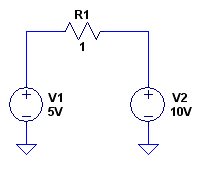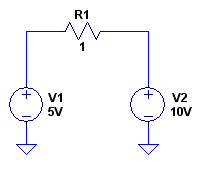# Power supply sinking current

I have this arrangement where 0.45V supply and 5v supply are connected to a load (say 6k resistor).
The 5V source is forcing current to flow into the 0.45V supply.

This is a bit counter intuitive. Power supplies usually source current.
Ckt schematic attached.

#### Attachments

•buffer prob.png
2.3 KB · Views: 445

vk6kro
That is a pretty standard Kirchoff Law problem.

Is this homework?

A hint would be to work out an equivalent resistor for all those resistors in parallel.

The 5V source is forcing current to flow into the 0.45V supply. This is a bit counter intuitive. Power supplies usually source current.

What is the magnitude and direction of the current here:That is a pretty standard Kirchoff Law problem.

Is this homework?

A hint would be to work out an equivalent resistor for all those resistors in parallel.

I wish it was a homework problem. I could have easily accepted current flowing in the other direction than what is expected.

The 0.45V is actually an opamp buffer output(unity gain, non-inverting), which sets the reference for 12 comparators. All the parallel resistors are hysteresis resistors of 12 comparators. Single supply comparator with Vcc=5v. Input at inverting input.
When the load is 0.1uF cap instead of the resistor R2, I get 0.7v across the capacitor.
If I replace the cap with a 1k resistor, I get 0.45v.
I can't figure out why the voltage of buffer is 0.7v when I connect a capacitor.

The capacitive load is not causing the voltage to be 0.7v. I have removed the capacitor and measured the output. It's still the same.

What is the magnitude and direction of the current here:5Amps flowing from right to left (10v to 5v).

vk6kro
There are many more components than are shown in the diagram and this includes many diode junctions inside the opamps. So, it gets difficult to predict where odd voltages are coming from.

However, it would be worth observing the voltages at various points with an oscilloscope to check for oscillation in some of the opamps.
This would explain some of the odd behaviour.

A voltage that is 0.45 volts across a resistor but 0.7 volts across a capacitor sounds familiar from power supply design, so it is possible you have some oscillation going on.

There are many more components than are shown in the diagram and this includes many diode junctions inside the opamps. So, it gets difficult to predict where odd voltages are coming from.

However, it would be worth observing the voltages at various points with an oscilloscope to check for oscillation in some of the opamps.
This would explain some of the odd behaviour.

A voltage that is 0.45 volts across a resistor but 0.7 volts across a capacitor sounds familiar from power supply design, so it is possible you have some oscillation going on.

All the voltages were measure using oscilloscope. I even put the scope in AC coupling to looks for oscillations. I saw none. The probe ground clip was as short as possible.

I also measured without any load across op amp output and ground (just the scope probe). No oscillations, but still 0.7v.